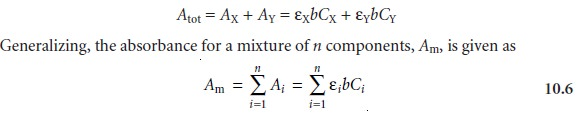Home | | Modern Analytical Chemistry | Absorbance and Concentration: Beer’s Law

# Absorbance and Concentration: Beer’s LawBeer’s Law and Multicomponent Samples

Absorbance and Concentration: Beer’s Law

When monochromatic electromagnetic radiation passes through an infinitesimally thin layer of sample, of thickness dx, it experiences a decrease in power of dP (Figure 10.21). The fractional decrease in power is proportional to the sample’s thick- ness and the analyte’s concentration, C; thus10.3where P is the power incident on the thin layer of sample, and α is a proportionality constant. Integrating the left side of equation 10.3 from P = P0 to P = PT, and the right side from x = 0 to x = b, where b is the sample’s overall thicknessConverting from ln to log, and substituting equation 10.2, gives

A = abC          …………. 10.4

where a is the analyte’s absorptivity with units of cm–1 conc–1. When concentration is expressed using molarity, the absorptivity is replaced by the molar absorptivity, ε (with units of cm–1 M–1)

A = εbC     …………. 10.5

The absorptivity and molar absorptivity give, in effect, the probability that the ana- lyte will absorb a photon of given energy. As a result, values for both a and ε depend on the wavelength of electromagnetic radiation.Equations 10.4 and 10.5, which establish the linear relationship between absorbance and concentration, are known as the Beer–Lambert law, or more commonly, as Beer’s law. Calibration curves based on Beer’s law are used routinely in quantitative analysis.

## Beer’s Law and Multicomponent Samples

Beer’s law can be extended to samples containing several absorbing components provided that there are no interactions between the components. Individual ab- sorbances, Ai, are additive. For a two-component mixture of X and Y, the total ab- sorbance, Atot, isStudy Material, Lecturing Notes, Assignment, Reference, Wiki description explanation, brief detail
Modern Analytical Chemistry: Spectroscopic Methods of Analysis : Absorbance and Concentration: Beer’s Law |